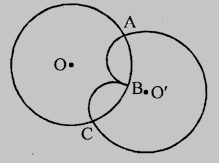# Prove that two different circles cannot intersect each other at more than two points.

To do:

We have to prove that two different circles cannot intersect each other at more than two points.

Solution:Let two circles intersect each other at three points $A, B$ and $C$

Two circles with centres $O$ and $O’$ intersect at $A, B$ and $C$

This implies,

$A, B$ and $C$ are non-collinear points.

Circle with centre $O$ passes through three points $A, B$ and $C$ and circle with centre $O’$ also passes through three points $A, B$ and $C$

One and only one circle can be drawn through three points.

Therefore, our supposition is wrong

Two circles cannot intersect each other not more than two points.

Updated on: 10-Oct-2022

182 Views